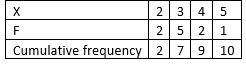### X 2 3 4 5 F 2 5 2 1   What is the median...

JAMB 2018

 X 2 3 4 5 F 2 5 2 1

What is the median of the distribution?

• A. 5
• B. 4
• C. 3
• D. 2
##### ExplanationWhen a group data is involved, cumulative frequency is used. The formula will be: Median=[(N+1)/2]th

For member of odd number items. That is, where N is odd

Median=([N/2]th + [N/2 + 1]th)/2

For member of odd number items. That is, where N is odd

Solution:

From the table, there are 20 members as indicated by the cumulative frequency. Since the members are odd (10) the median age will be ([N/2]th + [N/2 + 1]th)/2

Median=([10/2]th + [10/2 + 1]th)/2

=(5th + 6th)/2

The 5th member is 3 and the 6th member is on 3

Median=(3 + 3)/2

=6/2

Median = 3